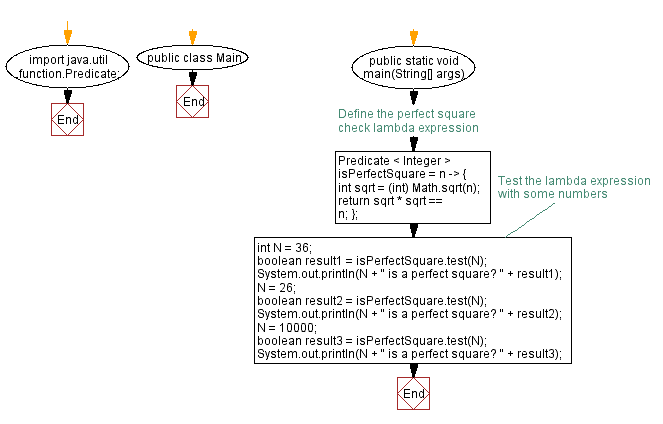# Java program to check if a number is a perfect square using lambda expression

## Java Lambda Program: Exercise-18 with Solution

Write a Java program to implement a lambda expression to check if a given number is a perfect square.

Note: A perfect square is an element of algebraic structure that is equal to the square of another element.

Sample Solution:

Java Code:

``````import java.util.function.Predicate;

public class Main {
public static void main(String[] args) {
// Define the perfect square check lambda expression
Predicate < Integer > isPerfectSquare = n -> {
int sqrt = (int) Math.sqrt(n);
return sqrt * sqrt == n;
};

// Test the lambda expression with some numbers
int N = 36;
boolean result1 = isPerfectSquare.test(N);
System.out.println(N + " is a perfect square? " + result1);

N = 26;
boolean result2 = isPerfectSquare.test(N);
System.out.println(N + " is a perfect square? " + result2);

N = 10000;
boolean result3 = isPerfectSquare.test(N);
System.out.println(N + " is a perfect square? " + result3);
}
}
``````

Sample Output:

```36 is a perfect square? true
26 is a perfect square? false
10000 is a perfect square? true
```

Explanation:

In the above exercise,

• Import the Predicate functional interface.
• In the main method, we define the lambda expression isPerfectSquare, which takes an integer N.
• The lambda expression checks if the square of the square root of n is equal to n. If it is, then N is a perfect square.
• We test the lambda expression by calling the test method with different numbers.
• Finally, we print the results to the console.

Flowchart:Live Demo:

Java Code Editor:

Improve this sample solution and post your code through Disqus

Java Lambda Exercises Previous: Java program to find length of longest and smallest string using lambda expression.
Java Lambda Exercises Next: Find second largest and smallest element in an Array.

What is the difficulty level of this exercise?

Test your Programming skills with w3resource's quiz.

﻿

## Java: Tips of the Day

How do I remove repeated elements from ArrayList?

If you don't want duplicates in a Collection, you should consider why you're using a Collection that allows duplicates. The easiest way to remove repeated elements is to add the contents to a Set (which will not allow duplicates) and then add the Set back to the ArrayList:

```Set<String> set = new HashSet<>(yourList);
yourList.clear();